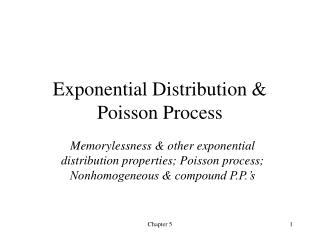# Exponential Distribution & Poisson Process - PowerPoint PPT PresentationDownload PresentationExponential Distribution & Poisson Process

Exponential Distribution & Poisson ProcessDownload Presentation## Exponential Distribution & Poisson Process

- - - - - - - - - - - - - - - - - - - - - - - - - - - E N D - - - - - - - - - - - - - - - - - - - - - - - - - - -
##### Presentation Transcript

1. Exponential Distribution & Poisson Process Memorylessness & other exponential distribution properties; Poisson process; Nonhomogeneous & compound P.P.’s Chapter 5

2. Exponential Distribution: Basic Facts • Density • CDF • MGF • Mean • Variance Coefficient of variation Chapter 5

3. Key Property: Memorylessness • Reliability: Amount of time a component has been in service has no effect on the amount of time until it fails • Inter-event times: Amount of time since the last event contains no information about the amount of time until the next event • Service times: Amount of remaining service time is independent of the amount of service time elapsed so far Chapter 5

4. Competing Exponentials: If X1 and X2 are independent exponential r.v.’s with parameters l1 and l2, resp., then (generalizes to any number of competing r.v.’s) Minimum of exponentials: If X1, X2 , …, Xn are independent exponential r.v.’s where Xn has parameter li, then min(X1, X2 , …, Xn) is exponential w/parameter l1 + l2 + … + ln Other Useful Properties Sum of n independent exponential r.v.’s with common parameter l has a gamma distribution w/parameters (n, l) Chapter 5

5. Counting Process A stochastic process {N(t), t  0} is a counting process if N(t) represents the total number of events that have occurred in [0, t] Then {N(t), t  0} must satisfy: N(t)  0 N(t) is an integer for all t If s < t, then N(s)  N(t) For s < t, N(t) - N(s) is the number of events that occur in the interval (s, t]. Chapter 5

6. Stationary & Independent Increments • A counting process has independent increments if, for any That is, the numbers of events that occur in nonoverlapping intervals are independent random variables. • A counting process has stationary increments if the distribution if, for any s < t, the distribution of N(t) – N(s) depends only on the length of the time interval, t – s. Chapter 5

7. Poisson Process Definition 1 A counting process {N(t), t  0} is a Poisson process with ratel, l > 0, if N(0) = 0 The process has independent increments The number of events in any interval of length t follows a Poisson distribution with mean lt (therefore, it has stationary increments), i.e., Chapter 5

8. Poisson Process Definition 2 A function f is said to be o(h) (“Little oh of h”) if A counting process {N(t), t  0} is a Poisson process with ratel, l > 0, if N(0) = 0 The process has stationary and independent increments Definitions 1 and 2 are equivalent! Chapter 5

9. Interarrival and Waiting Times N(t) The times between arrivals T1, T2, … are independent exponential r.v.’s with mean 1/l: The (total) waiting time until the nth event has a gamma dist’n: S1 S2 S3 S4 t T1 T2 T3 T4 Chapter 5

10. Other Poisson Process Properties Poisson Splitting: Suppose {N(t), t  0} is a P.P. with rate l, and suppose that each time an event occurs, it is classified as type I with probability p and type II with probability 1-p, independently of all other events. Let N1(t) and N2(t), respectively, be the number of type I and type II events up to time t. Then {N1(t), t  0} and {N2(t), t  0} are independent Poisson processes with respective rates lp and l(1-p). Chapter 5

11. Other Poisson Process Properties Competing Poisson Processes: Suppose {N1(t), t  0} and {N2(t), t  0} are independent Poisson processes with respective rates l1 and l2. Let Sni be the time of the nth event of process i, i = 1,2. Chapter 5

12. Other Poisson Process Properties If Y1, Y2, …, Yn are random variables, then Y(1), Y(2), …, Y(n) are their order statistics if Y(k) is the kth smallest value among Y1, Y2, …, Yn, k = 1, …, n. Conditional Distribution of Arrival Times: Suppose {N(t), t  0} is a Poisson process with rate l and for some time t we know that N(t) = n. Then the arrival times S1, S2, …, Sn have the same conditional distribution as the order statistics of n independent uniform random variables on (0, t). Chapter 5

13. Nonhomogeneous Poisson Process A counting process {N(t), t  0} is a nonhomogeneousPoisson process with intensity functionl(t), t 0, if: N(0) = 0 The process has independent increments (not stationary incr.) Let Then Chapter 5

14. Compound Poisson Process A counting process {X(t), t  0} is a compoundPoisson process if: where {N(t), t  0} is a Poisson process and {Yi, i = 1, 2, …} are independent, identically distributed r.v.’s that are independent of {N(t), t  0}. By conditioning on N(t), we can obtain: Chapter 5